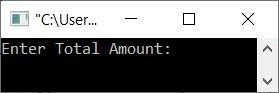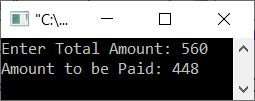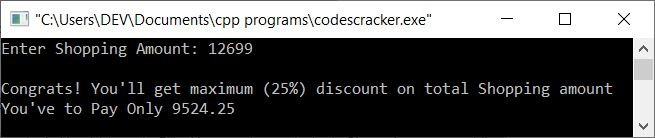# C++ program to calculate the price after applying the discount

This article contains a C++ program that will calculate and print the price to be paid after applying any applicable discounts based on the total purchase amount done or entered by the user (at run-time). The discount must be offered based on the total shopping amount as shown in the table below:

Total Shopping Amount Discount
<=100 No discount
>100 & <=200 5%
>200 & <=400 10%
>400 & <=800 20%
>800 25%

## Find and print the price to be paid after applying the discount

The question is: write a program in C++ to find and print the price that has to be paid after applying the discount. The program given below is the answer to this question:

```#include<iostream>

using namespace std;
int main()
{
float amount, discount, topaid;
cout<<"Enter Total Amount: ";
cin>>amount;
if(amount<=100)
cout<<"Amount to be Paid: "<<amount;
else
{
if(amount>100 && amount<=200)
{
discount = (amount*5)/100;
topaid = amount-discount;
}
else if(amount>200 && amount<=400)
{
discount = (amount*10)/100;
topaid = amount-discount;
}
else if(amount>400 && amount<=800)
{
discount = (amount*20)/100;
topaid = amount-discount;
}
else
{
discount = (amount*25)/100;
topaid = amount-discount;
}
cout<<"Amount to be Paid: "<<topaid;
}
cout<<endl;
return 0;
}```

The snapshot given below shows the initial output produced by the above C++ program on calculating the price (amount to be paid) after subtracting the discount (if any):Now enter the total purchase amount, say \$560, and press the ENTER key to calculate and print the price that has to be paid after applying the discount, as shown in the snapshot given below:In the above program, I've applied the condition amount<=100 using if, which checks whether the purchase amount is less than or equal to 100 or not. If the purchase amount is \$100 or less, then there is no discount to apply. As a result, I printed it exactly as is, with the amount of shopping the customer did.

Otherwise, if the condition evaluates to false, the program flow goes to else's body, and inside the else, I've created a total of three conditions to check and apply the discount, based on the discount given in the table earlier in this article. In this way, the program is created.

Let's modify the above program to provide a good user experience by displaying the discount (if any) applied to his or her purchase:

```#include<iostream>

using namespace std;
int main()
{
float amount, discount, topaid;
cout<<"Enter Shopping Amount: ";
cin>>amount;
if(amount<=100)
{
cout<<"\nSorry! No discount for shopping under 100";
cout<<"You've to Pay "<<amount;
}
else
{
if(amount>100 && amount<=200)
{
cout<<"\nCongrats! You'll get 5% discount on total Shopping amount";
discount = (amount*5)/100;
topaid = amount-discount;
}
else if(amount>200 && amount<=400)
{
cout<<"\nCongrats! You'll get 10% discount on total Shopping amount";
discount = (amount*10)/100;
topaid = amount-discount;
}
else if(amount>400 && amount<=800)
{
cout<<"\nCongrats! You'll get 20% discount on total Shopping amount";
discount = (amount*20)/100;
topaid = amount-discount;
}
else
{
cout<<"\nCongrats! You'll get maximum (25%) discount on total Shopping amount";
discount = (amount*25)/100;
topaid = amount-discount;
}
cout<<"\nYou've to Pay Only "<<topaid;
}
cout<<endl;
return 0;
}```

Here is its sample run with user input of 12699 as the total shopping amount:C++ Quiz

« Previous Program Next Program »